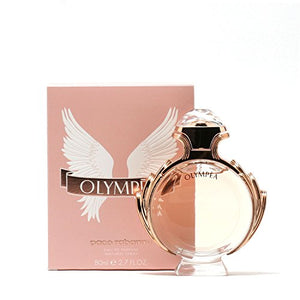# Paco Rabanne Olympea Ladies - Edp Spray 2.7 Oz

• \$143.20

• T

• o

• p

• N

• o

• t

• e

• s

• :

• g

• r

• e

• e

• n

• m

• a

• n

• d

• a

• r

• i

• n

• ,

• w

• a

• t

• e

• r

• j

• a

• s

• m

• i

• n

• e

• ,

• g

• i

• n

• g

• e

• r

• l

• i

• l

• y

• M

• i

• d

• d

• l

• e

• N

• o

• t

• e

• s

• :

• s

• a

• l

• t

• e

• d

• v

• a

• n

• i

• l

• l

• a

• B

• a

• s

• e

• N

• o

• t

• e

• s

• :

• s

• a

• n

• d

• a

• l

• w

• o

• o

• d

• ,

• c

• a

• s

• h

• m

• e

• r

• e

• a

• n

• d

• a

• m

• b

• e

• r

• g

• r

• i

• s

• .

• Size : 2.7 oz

Olympea by Paco Rabanne is an oriental floral scent for women. It features top notes of green mandarin, water jasmine and ginger lily. Middle note is salted vanilla. Base notes are sandalwood, cashmere and ambergris. This fragrance is the perfect blend of salty and sweet!

every pages